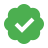# Supervisely/ Precision and RecallCustom Updated An hour ago Free
Takes 2 (or 1) projects as input, for each pair of classes calculates Precision and Recall and their mean values for all defined classes at a given Intersection over Union (IoU) threshold

# Precision and Recall for object detection and instance segmentation.

A key role in calculating metrics for object detection and instance segmentation tasks is played by Intersection over Union(IoU). You can read more about IoU in our other guide(!add link). Here we will briefly describe it.

### Intersection Over Union (IOU)

Intersection Over Union (IOU) is measure based on Jaccard Index that evaluates the overlap between two bounding boxes or instance segments. It requires a ground truth bounding box(instance segment) Bgt and a predicted bounding box(instance segment) Bp.By applying the IOU we can tell if a detection is valid (True Positive) or not (False Positive).
IOU is given by the overlapping area between the predicted instance and the ground truth instance divided by the area of union between them:Some extra basic concepts that we will use:

• True Positive (TP): A correct detection. Detection with IOU ≥ threshold• False Positive (FP): A wrong detection. Detection with IOU < threshold• False Negative (FN): A ground truth not detected• True Negative (TN): Does not apply. It would represent a corrected misdetection. In the object detection task there are many possible bounding boxes that should not be detected within an image. Thus, TN would be all possible bounding boxes that were correctly not detected (so many possible boxes within an image). That's why it is not used by the metrics.

threshold - it is usually set to 0.5, 0.75 or swept from 0.5 to 0.95.

### Precision

Precision is the ability of a model to identify only the relevant objects. It is the percentage of correct positive predictions and is given by:### Recall

Recall is the ability of a model to find all the relevant cases (all ground truth bounding boxes). It is the percentage of true positive detected among all relevant ground truths and is given by:## Config example

``````{
"iou": 0.5,
"project_1": "project_1_name",
"project_2": "project_2_name",
"classes_mapping": {
"pr1_cls_name_1": "pr2_cls_name_1",
"pr1_cls_name_2": "pr2_cls_name_2"
}
}
``````

Here:

1. "iou" - Intersection over Union threshold.
2. “project_1” - name of the first project involved in IoU evalutaion.
3. “project_2” - name of the second project involved in IoU evaluation. In case you want to evaluate metric between objects of the same project, you can simply delete this field from config.
4. “classes_mapping” - field matches pairs of objects classes between which IoU will be evaluated.

### Docker Image

`supervisely/metric-precrec:latest`

ID
75278
Version ID
84510
First released
5 months ago
Last updated
An hour ago

s